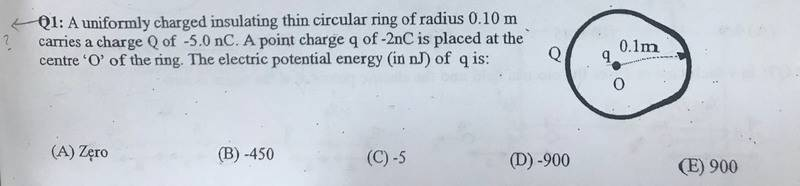# Find the electric potential energy

## Homework Statement## Homework Equations

##V= \frac {kq}{r}##
##V= \frac {U}{q}##

## The Attempt at a Solution

##V= \frac {kq}{r}## = 9*109*-5*10-9 /0.1= -450 V
##U= \frac {V}{q}## = -450 * -2*10-9 = 900nJ

gneill
Mentor

## Homework Statement

View attachment 223345

## Homework Equations

##V= \frac {kq}{r}##
##V= \frac {U}{q}##

## The Attempt at a Solution

##V= \frac {kq}{r}## = 9*109*-5*10-9 /0.1= -450 V
##U= \frac {V}{q}## = -450 * -2*10-9 = 900nJ
Yes, but the form of your second relevant equation in your last line of calculations is not. Fortunately you did the actual calculation correctly anyways•